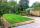# Right triangle eq2

Find the lengths of the sides and the angles in the right triangle. Given area S = 210 and perimeter o = 70.

Result

a =  21
b =  20
c =  29
A =  46.397 °
B =  43.603 °
C =  90 °

#### Solution:Checkout calculation with our calculator of quadratic equations.

Try calculation via our triangle calculator.Leave us a comment of example and its solution (i.e. if it is still somewhat unclear...):

Showing 0 comments:Be the first to comment!#### To solve this example are needed these knowledge from mathematics:

Looking for help with calculating roots of a quadratic equation? Do you have a linear equation or system of equations and looking for its solution? Or do you have quadratic equation? Pythagorean theorem is the base for the right triangle calculator. Most natural application of trigonometry and trigonometric functions is a calculation of the triangles. Common and less common calculations of different types of triangles offers our triangle calculator. Word trigonometry comes from Greek and literally means triangle calculation.

## Next similar examples:

1. Isosceles triangleThe perimeter of an isosceles triangle is 112 cm. The length of the arm to the length of the base is at ratio 5:6. Find the triangle area.
2. Right triangle AlefThe obvod of a right triangle is 84 cm, the hypotenuse is 37 cm long. Determine the lengths of the legs.
3. RT leg and perimeterCalculate the length of the sides of a right triangle ABC with hypotenuse c when the length of a leg a= 84 and perimeter of the triangle o = 269.
4. Triangle - is RT?Triangle has a circumference of 90 cm. Side b is 1 cm longer than c, side c is 31 cm longer than side a. Calculate the length of sides and determine whether triangle is a right triangle.
5. Triangle ABCIn a triangle ABC side b measure 10 cm less than the side a and side b is half of the side c. Calculate the length of sides if the circumference of the triangle is 42 cm.
6. ParallelogramParallelogram has sides lengths in the ratio 3: 4 and perimeter 2.8 meters. Determine the lengths of the sides.
7. ParallelogramThe perimeter of the parallelogram is 417 cm. The length of one side is 1.7-times longer than the length of the shorter side. What is the length of sides of a parallelogram?
8. Two squaresTwo squares whose sides are in the ratio 5:2 have sum of its perimeters 73 cm. Calculate the sum of area this two squares.
9. Rectangle - sides ratioCalculate area of rectangle whose sides are in ratio 3:13 and perimeter is 673.
10. Circle arcCircle segment has a circumference of 135.26 dm and 2096.58 dm2 area. Calculate the radius of the circle and size of central angle.
11. PropertyThe length of the rectangle-shaped property is 8 meters less than three times of the width. If we increase the width 5% of a length and lendth reduce by 14% of the width it will increase the property perimeter by 13 meters. How much will the property cost
12. Rectangle SSPerimeter of a rectangle is 268 cm and its diagonal is 99.3 cm. Determine the dimensions of the rectangle.
13. PlotThe length of the rectangle is 8 smaller than three times the width. If we increase the width by 5% of the length and the length is reduced by 14% of the width, the circumference of rectangle will be increased by 30 m. What are the dimensions of the rectan
14. RectangleThe width of the rectangle is 65% of its length. Perimeter of the rectangle is 132 cm. Determine the dimensions of the rectangle.
15. GardenThe garden has a rectangular shape and has a circumference of 130 m and area 800.25 m2. Calculate the dimensions of the garden.
16. GardensThe garden has the square shape with circumference 124 m. Divide it into two rectangular gardens, one should circumference 10 meters more than the second. What size will have a gardens?
17. Rectangle from stringString 12m. Make rectangle when one side is two times longer than its width.